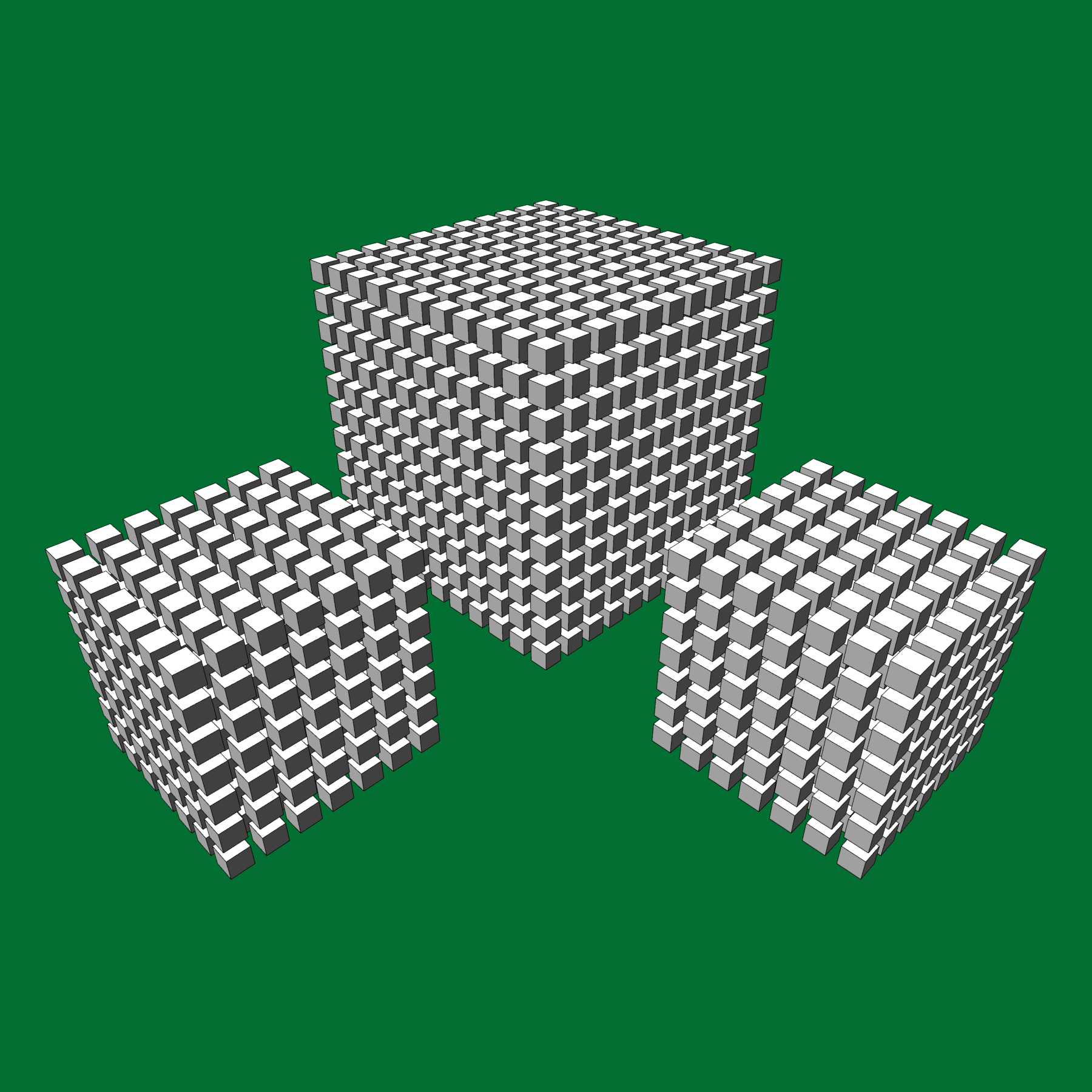2017 = 7³ + 7³ + 11³ Digital Print 2016

The number 2017 can be expressed as the sum of three cubes 7³ + 7³ + 11³. It remains an open problem to determine which integers can be expressed as a sum of three cubes.

### Background and Inspiration

This work was originally done for use as cover art for the February 2017 issue of Mathematics Magazine (the first issue of that year) to celebrate the year 2017. This is the eleventh of 25 original artworks I created for the journal Mathematics Magazine during 2015–2019.

A problem was posed in 1954 to find integers solutions to the equation $$a^3 + b^3 + c^3 = n$$ for values of $$n$$ between 1 and 100. No solutions are possible when $$n$$ is congruent to $$4$$ or $$5$$ modulo $$9$$ (4, 5, 13, 14, $$\ldots$$). Negative values are allowed for $$a$$, $$b$$, and $$c$$. It remains an open problem if every integer not congruent to $$4$$ or $$5$$ modulo $$9$$ can be expressed as a sum of three cubes.

At the time I created this piece, 33 was the smallest positive integer where no solution was known according to the paper Newer sums of three cubes. The author stated there were 13 values of $$n$$ less than 1000 for which no solutions had been found: 33, 42, 114, 165,390, 579,627, 633, 732, 795, 906, 921, and 975.

In 2019, solutions for $$n=33$$ and $$n = 42$$ were discovered. $$42 = (-80538738812075974)^3 + 80435758145817515 ^3+ 12602123297335631^3$$ Also a new way of representing 3 was discovered along with a way of representing 906. $$569936821221962380720^3 + (-569936821113563493509)^3 + (-472715493453327032)^3 = 3$$

### Publication History

• Mathematics Magazine, Cover Art, Vol. 90, No. 1, February 2017.